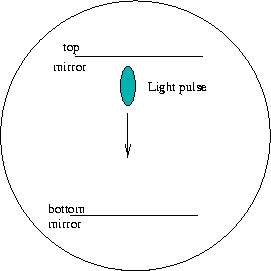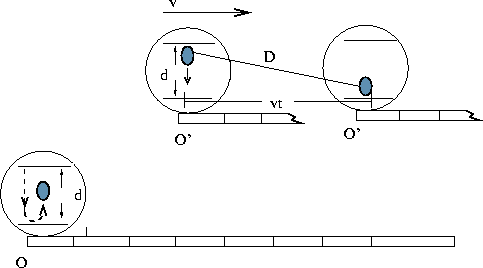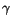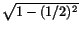Next: Length Contraction Up: Special Relativity Previous: Frames of Reference and

# Time Dilation

The Galilean transformation laws 11.1-11.3 were derived by applying common sense to simple thought experiments. We now need to abandon common sense, to some extent, but retain the speed of light postulate. Surprisingly, we are almost immediately forced to abandon the notion of absolute time (i.e. Galilean transformation law 11.2). To see how this comes about, imagine constructing a very simple clock that consists of light pulses being bouncing back and forth between two vertical mirrors, as shown in Fig. 11.4 below.The distance d between the mirrors is 300 million meters, so that it takes precisely one second for light to get from one mirror to another. (Clearly this is another gedanken experiment: easy to imagine, but hard to do in practice.) The clock therefore ticks one second everytime a light pulse hits one of the mirrors. Suppose now that you have two identical such clocks, one in frame of reference O, the other in O', moving at relative speed v, as illustrated in Fig.11.5 below.Let us now synchronize the two clocks so that the light pulses in both clocks leave the top mirror at precisely t = t' = 0. When the light pulse in O'arrives at the bottom mirror this clock tick once so that this time interval is one second, as measured by O'. Now we wish to find out what is time does the clock in O read at the instant that the clock in O' ticks once second? The intuitive answer is that if one second passes in O' then one second must also pass in O. However, we need to dispense with our intuition. By the time the light pulse in O'arrives at the bottom mirror, the clock in O' has moved forward a horizontal distance of v x t relative to O, where t is the time that measured by the clock in O. An observer in Otherefore sees this light pulse move along the diagonal line shown in the Fig. 11.5. The total distance D traveled by this light pulse, i.e. the length of the diagonal, is necessarily greater than d, the distance between the mirrors.

We now invoke the speed of light postulate, which requires the light pulses in both clocks to move at precisely the same speed relative to O and hence travel the same distance in the given time interval. Hence, the light pulse in the clock attached to O also has traveled a distance D, and has already bounced off the bottom mirror by the time the clock in O' ticks one second. An observer in O therefore concludes that the clock attached to O' is running slow.

This is a very startling result. It says that two identical clocks that run at precisely the same rate when compared in the same reference frame will appear to disagree when viewed from different frames: time is not absolute, but depends on the frame of reference of the observer. This conclusion seems even more bizarre if one recalls that the relativity of motion postulate does not allow us to discern which of the two observers is actually in motion. If we repeat the above argument, but from the point of view of an observer moving with O' , we will be lead to precisely the same conclusion, only in reverse. An observer in O' sees the light pulse of the clock in O travels along a diagonal Dand is forced to conclude that the clock in O is running slowly. Each observer therefore thinks that it is the other clock that is running slowly, and there is no sense in which we can claim that one clock or the other is the culprit. Time itself is relative.

It is not difficult to get a quantitative expression relating the time elapsed on O's clock to that of O'. The distance between the mirrors, dis related to the time t' measure by O' according to d = ct', where c, as usual is the speed of light. (We are using the fact that displacement = (velocity x time).) Since the speed of light is precisely the same for both observers, the time t elapsed in that frame is related to the distance D traveled relative to O by a similar relation: D = ct. Using the Pythagorean theorem relating the sides of a right triangle, we know that D2 = d2 + (vt)2. When we substitute the expressions for D and d in terms of the corresponding times, we get the desired relationship:

 (ct)2 = (ct')2 + (vt)2 (11.4)

With a little bit of algebra we can express t explicitly in terms of t'. What we find is that the time measured in O is proportional to the time as measured by in O':

 t =t' (11.5)

The constant of proportionality,is called the time dilation factor. It depends on the relative velocity v and is given by the expression:=(11.6)

There are several important things to notice about the time dilation factor. First of all, no matter what the velocity is,is always greater than one, so the clocks always appears to run slower when observed by someone in a different (uniformly moving frame.

Secondly,depends only on (v/c)2, the square of the ratio of the relative velocity v to the speed of light c. Hence it doesn't care about whether v is positive or negative (i.e. whether the motion is to the right or to the left). Moreover, when v is much less than c, the ratio v2/c2 is much less then one. In this case the denominator in 11.6 is very nearly equal to one, and so is. This explains why the bizarre consequences of Special Relativity do not affect our daily lives. We do not regularly experience relative velocities anywhere near 300 million meters per second. At the more Earthly speed of 100 km/hr, or about 30 m/s, the ratio v/c is 30 divided by 30 million, or one millionth. The square of a very small number is smaller still. In this case v2/c2 = 10-12and consequently, the time dilation factor differs from one by only one part in 1012. This is clearly too small to be detectable. We can now understand why many of the predictions of special relativity are counter-intuitive. Our intuition is based on low velocity experiences. If there is a life form somewhere in the Universe that regularly travels at near light speeds, its intuition would lead to very different conclusions.

The time dilation factor is only relevant when the relative velocity is a significant fraction of the speed of light. For example, if v = c/2 (i.e. one half the speed of light), the time dilation factor is 1/or approximately 1.2. In this case an observer in O sees the clock in O' run 20 % slower. Conversely, an observer moving with O' will see the clock in O running 20% slower.

One final important feature of the time dilation factor is that as the relative velocity gets near the speed of light, the denominator nears zero, and expression forblows up: dividing by zero gives infinity. This suggests that something strange happens as velocities approach the speed of light. We will have more to say about this a bit later.

To understand the true significance of all this, one must keep in mind that time dilation does not apply only to the special types of clocks I described above. In fact, all physical processes appear to slow down when view from a different uniformly moving frame. This effect has been verified experimentally in many contexts. One of these involves elementary particles called muons, which are created when high energy cosmic rays hit the upper atmosphere. These muons are unstable and normally decay into a different form of matter within about two microseconds (two millionths of a second). The muons created by cosmic rays plummet towards the Earth at very high speeds, typically of the order of 99% of the speed of light. At this speed they should be able to travel a distance of (300 million meters/second times .000002 seconds ) or 600 meters. Hence they should decay somewhere in the upper atmosphere. What is observed, however, is that the muons get significantly closer to the Earth's surface than the above argument suggests. In fact, some of them hit the Earth.

The explanation comes from Special Relativity. In accordance with the time dilation effect, the physical process responsible for muon decay appears to be running slowly when viewed from the Earth. If the muon is moving at a velocity of .99c, the time dilation factoris about 7. Thus, to an observer on Earth, the muon appears to live seven times longer (about 14 microseconds instead of just 2). It therefore can travel seven times further (over 4 kilometers), and traverses most of the atmosphere before decaying.Next: Length Contraction Up: Special Relativity Previous: Frames of Reference and
modtech@theory.uwinnipeg.ca
1999-09-29